## Taylor Series

A Taylor series is a series expansion of a Function about a point. A 1-D Taylor series is an expansion of a Scalar Function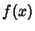about a point. If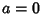, the expansion is known as a Maclaurin Series.(1)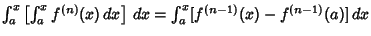(2)
Continuing,(3)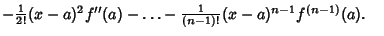(4)
Therefore, we obtain the 1-D Taylor series(5)
whereis a remainder term defined by(6)

Using the Mean-Value Theorem for a function, it must be true that(7)

for some. Therefore, integratingtimes gives the result(8)

The maximum error is then the maximum value of (8) for all possible.

An alternative form of the 1-D Taylor series may be obtained by letting(9)

so that(10)

Substitute this result into (5) to give(11)

A Taylor series of a Function in two variables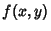is given by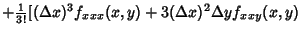(12)

This can be further generalized for a Function invariables,(13)

Rewriting,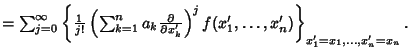(14)

Takingin (13) gives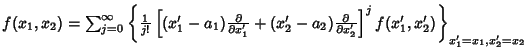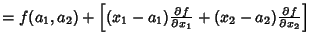(15)

Takingin (14) gives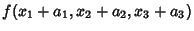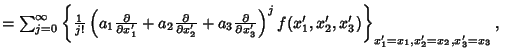(16)
or, in Vector form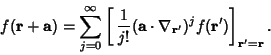(17)

The zeroth- and first-order terms are(18)

and(19)

respectively. The second-order term is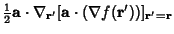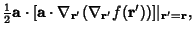(20)

so the first few terms of the expansion are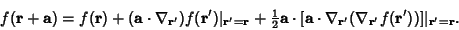(21)

Taylor series can also be defined for functions of a Complex variable. By the Cauchy Integral Formula,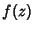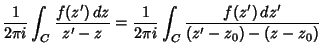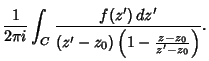(22)

In the interior of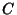,(23)

so, using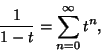(24)

it follows that(25)

Using the Cauchy Integral Formula for derivatives,(26)

See also Cauchy Remainder Form, Lagrange Expansion, Laurent Series, Legendre Series, Maclaurin Series, Newton's Forward Difference Formula

References

Abramowitz, M. and Stegun, C. A. (Eds.). Handbook of Mathematical Functions with Formulas, Graphs, and Mathematical Tables, 9th printing. New York: Dover, p. 880, 1972.

Arfken, G. Taylor's Expansion.'' §5.6 in Mathematical Methods for Physicists, 3rd ed. Orlando, FL: Academic Press, pp. 303-313, 1985.

Morse, P. M. and Feshbach, H. Derivatives of Analytic Functions, Taylor and Laurent Series.'' §4.3 in Methods of Theoretical Physics, Part I. New York: McGraw-Hill, pp. 374-398, 1953.